# Maharashtra Board Class 9 Maths Solutions Chapter 8 Trigonometry Practice Set 8.2

## Maharashtra State Board Class 9 Maths Solutions Chapter 8 Trigonometry Practice Set 8.2

Question 1.
In the following table, a ratio is given in each column. Find the remaining two ratios in the column and complete the table.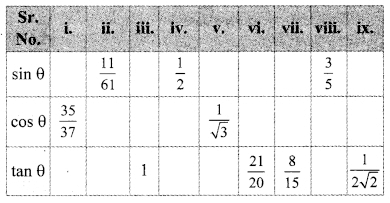Solution:
i. cos θ = $$\frac { 35 }{ 37 }$$ …(i) )[Given]
In right angled ∆ABC,∠C = θ.Let the common multiple be k.
∴ BC = 35k and AC = 37k
Now, AC2 = AB2 + BC2 …[Pythagoras theorem]
∴ (37k)2 = AB2+ (35k)2
1369k2 = AB2 + 1225k2
AB2 = 1369k2 – 1225k2
= 144k2
AB = 144k2
AB = $$\sqrt { 2ghK }$$2 … [Taking square root of both sides]
= 12kii. sin θ = $$\frac { 11 }{ 61 }$$ …..(i) [Given]
In right angled ∆ABC, ∠C = θ.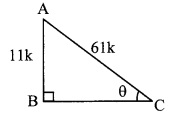Let the common multiple be k.
AB = 11k and AC = 61k
Now, AC2 = AB2 + BC2 …[Pythagoras theorem]
∴ (61k)2 = (11k)2 + BC2
∴ 3721k2 = 121k2 + BC2
∴ BC2 = 3721k2 – 121k2 = 3600k2
BC = $$\sqrt { 3600{ k }^{ 2 } }$$ .. .[Taking square root of both sides]
= 60k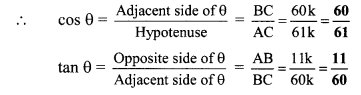iii. tan θ = 1 = $$\frac { 1 }{ 1 }$$ ..(i) [Given]
In right angled ∆ABC,
∠C = θ.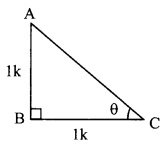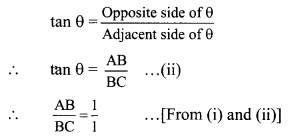Let the common multiple be k.
∴ AB = 1k and BC = 1k
Now, AC2 = AB2 + BC2 …[Pythagoras theorem]
= K2 + K2
= 2K2
∴ AC = $$\sqrt { 2{ k }$$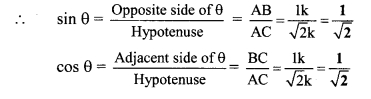iv. sin θ = $$\frac { 1 }{ 2 }$$ ..(i) [Given]
In right angled ∆ABC,
∠C = θ.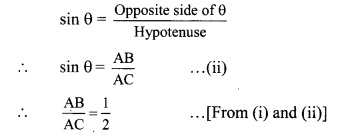Let the common multiple be k.
∴ AB = 1k and BC = 2k
Now, AC2 = AB2 + BC2 …[Pythagoras theorem]
∴ 2K2 = K2 + BC2
∴ 4K2 = K2 + BC2
∴ BC2 = 4K2 – K2 = 3K2
∴ BC = $$\sqrt { 3{ k }^{ 2 } }$$ .. .[Taking square root of both sides]
= $$\sqrt { 3{ k }$$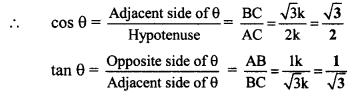v. cos θ = $$\frac { 1 }{ \sqrt { 3 } }$$ ..(i) [Given]
In right angled ∆ABC,
∠C = θ.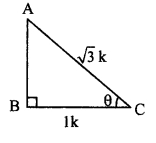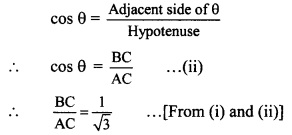Let the common multiple be k.
∴ AB = 1k and BC = √3k
Now, AC2 = AB2 + BC2 …[Pythagoras theorem]
∴ (√3K)2 = AB2 + K2
∴ 3K2 = 3K2 – K2 = 2K2
∴ AB = $$\sqrt { 2{ k }^{ 2 } }$$ .. .[Taking square root of both sides]
AB = √2K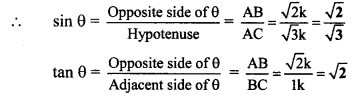vi. cos θ = $$\frac { 21 }{ \sqrt { 20 } }$$ ..(i) [Given]
In right angled ∆ABC,
∠C = θ.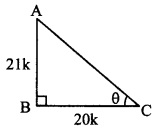Let the common multiple be k.
∴ AB = 21k and BC = 20k
Now, AC2 = AB2 + BC2 …[Pythagoras theorem]
= (21)K2 + (20K)2
= 441K2 – 4002
= 841K2
∴ AB = $$\sqrt { 841{ k }^{ 2 } }$$ .. .[Taking square root of both sides]
= 29Kvii. tan θ = $$\frac { 8 }{ 15 }$$ ..(i) [Given]
In right angled ∆ABC,
∠C = θ.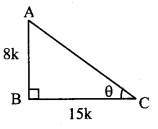Let the common multiple be k.
∴ AB = 8k and BC = 15k
Now, AC2 = AB2 + BC2 …[Pythagoras theorem]
= (8)K2 + (15K)2
= 64K2 – 2252
= 289K2
∴ AC = $$\sqrt { 289{ k }^{ 2 } }$$ .. .[Taking square root of both sides]
= 17Kviii. sin θ = $$\frac { 3 }{ 5 }$$ ..(i) [Given]
In right angled ∆ABC,
∠C = θ.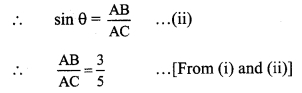Let the common multiple be k.
∴ AB = 3k and AC = 5k
Now, AC2 = AB2 + BC2 …[Pythagoras theorem]
∴ (5)K2= (3)K2 + BC2
∴ 25K2 = 9K2 – 2252
∴ BC2 = 25K2 – 9K2
∴ BC = $$\sqrt { 16{ k }^{ 2 } }$$ .. .[Taking square root of both sides]
= 4K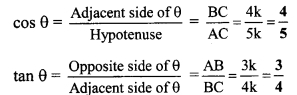ix. tan θ = $$\frac { 1 }{ 2\sqrt { 2 } }$$ ..(i) [Given]
In right angled ∆ABC,
∠C = θ.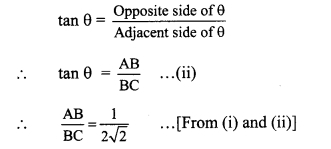Let the common multiple be k.
∴ AB = 1k and AC = 2√2 k
Now, AC2 = AB2 + BC2 …[Pythagoras theorem]
= K2 + (2√2 k )2
= K2 – 2252
= 25K2 + 8K2
= 9K2
∴ AC = $$\sqrt { 9{ k }^{ 2 } }$$ .. .[Taking square root of both sides]
= 3K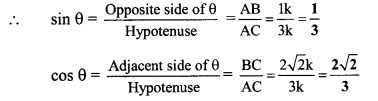Question 2.
Find the values of:
i. 5 sin 30° + 3 tan 45°
ii. $$\frac { 4 }{ 5 }$$tan2 60° + 3 sin2 60°
iii. 2 sin 30° + cos 0° + 3 sin 90°
iv. $$\frac{\tan 60^{\circ}}{\sin 60^{\circ}+\cos 60^{\circ}}$$
v. cos2 45° + sin2 30°
vi. cos 60° x cos 30° + sin 60° x sin 30°
Solution:
i. sin 30° = $$\frac { 1 }{ 2 }$$ and tan 45° = 1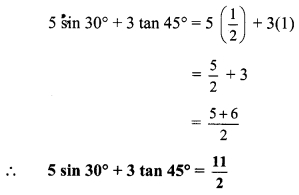ii. $$\frac { 4 }{ 5 }$$tan2 60° + 3 sin2 60°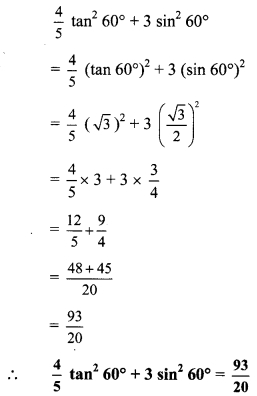iii. 2 sin 30° + cos 0° + 3 sin 90°
2 sin 30° + cos0° + 3 sin 90° = 2 ($$\frac { 1 }{ 2 }$$) + 1 + 3(1)
= 1 + 1 + 3
∴ 2 sin 30° + cos 0° + 3 sin 90° = 5

iv. $$\frac{\tan 60^{\circ}}{\sin 60^{\circ}+\cos 60^{\circ}}$$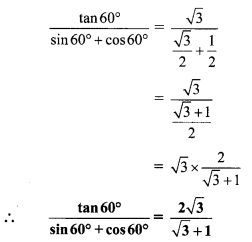v. cos2 45° + sin2 30°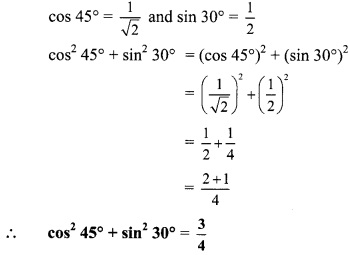vi. cos 60° x cos 30° + sin 60° x sin 30°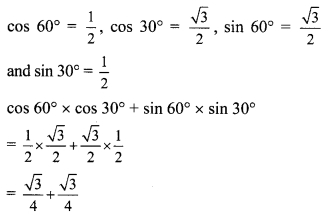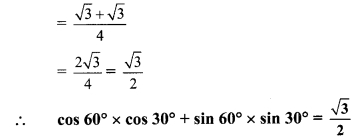Question 3.
If sin θ = $$\frac { 4 }{ 5 }$$ , then find cos θ.
Solution:
sin θ = $$\frac { 4 }{ 5 }$$ .. .(i)[Given]
In right angled ∆ABC,
∠C = θ.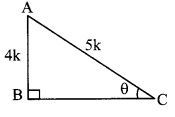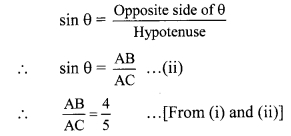Let the common multiple be k.
∴ AB = 4k and AC = 5k
Now, AC2 = AB2 + BC2 … [Pythagoras theorem]
∴ (5 k)2 = (4k)2 + BC2
∴ 25k2 = 16k2 + BC2
∴ BC2 = 25k2 – 16k2 = 9k2
∴ BC = $$\sqrt { 9{ k }^{ 2 } }$$ . .[Taking square root of both sides]
= 3kQuestion 4.
If cos θ = $$\frac { 15 }{ 17 }$$ , then find sin θ.
Solution:
cos θ = $$\frac { 15 }{ 17 }$$ .. .(i)[Given]
In right angled ∆ABC,
∠C = θ.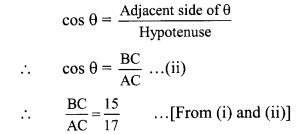Let the common multiple be k.
∴ BC = 15k and AC = 17k
Now, AC2 = AB2 + BC2 … [Pythagoras theorem]
∴ (17 k)2 = AB2 + (15K)2
∴ 289k2 = AB2 + 2252
∴ AB2 = 289k2 – 225k2
= 64k2
∴ AB = $$\sqrt { 64{ k }^{ 2 } }$$ . .[Taking square root of both sides]
= 8k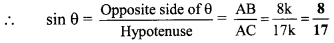Maharashtra Board Class 9 Maths Chapter 8 Trigonometry Practice Set 8.2 Intext Questions and Activities

Question 1.
In right angled ∆PQR, ∠Q = 900. Therefore ∠P and ∠R are complementary angles of each other. Verify the following ratios.
i. sin θ = cos (90 – θ)
ii. cos θ = sin (90 – θ)
iii. sin 30° = cos (90° – 30°) = cos 60°
iv. cos 30° = sin (90° – 30°) = sin 60° (Textbook pg. no. 107)
Solution:
In ∆PQR, ∠Q = 90°, ∠P = θ
∴ ∠R = 90 – θi. sin θ = cos (90 – θ)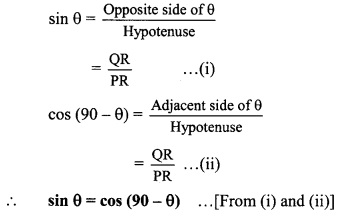ii. cos θ = sin (90 – θ)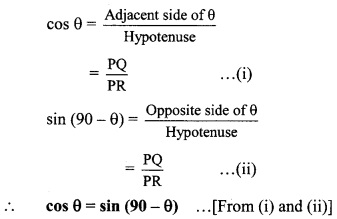iii. Let ∠P = θ = 30°
∴ ∠R = 90° – 30°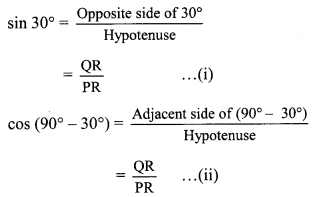sin 30° = cos (90° – 30°) … [From (i) and (ii)]
sin 30° = cos 60°

iv. cos 30° = sin (90° – 30°) = sin 60°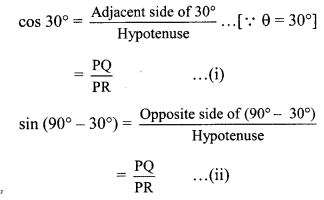∴ cos 30° = sin (90° – 30°) .,.[From (i) and (ii)]
∴ cos 30° = sin 60°

Question 2.
In right angled ∆PQR, ∠Q = 90°, ∠R = θ and if sin θ = $$\frac { 5 }{ 13 }$$, then find cos θ and tan θ. (Textbook pg. no. 110)
Solution:
i. Take the given trigonometric ratio as 13k equation (i).
sin θ = $$\frac { 5 }{ 13 }$$ .. .(i)[Given]
By using the definition write the trigonometric ratio of sin O and take it as equation (ii).
In right angled ∆PQR, ∠R = θ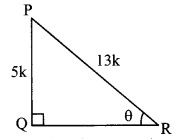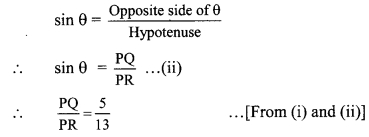Let the common multiple be k.
∴ PQ = 5k and PR = 13k
Find QR by using Pythagoras theorem.
PR2 = PQ2 + QR2 … [Pythagoras theorem]
∴ (13k)2 = (5k)2 + QR2
∴ 169k2 = 25k2 + QR2
∴ QR2 = 169k2 – 25k2
= 144k2
∴ QR = $$\sqrt { 144{ k }^{ 2 } }$$ . . . [Taking square root of both sides]
= 12kQuestion 3.
While solving the above Illustrative example, why the lengths of PQ and PR are taken 5k and 13k? (Textbook pg. no. 111)
Solution:
$$\frac { PQ }{ PR }$$ = $$\frac { 5 }{ 13 }$$ … [Given]
Here, the ratio of the lengths of sides PQ and PR is 5 : 13.
The actual lengths of the sides can be any multiple of the ratio. Hence, we consider the multiple k while solving.

Question 4.
While solving the above illustrative example, can we take the lengths of PQ and PR as 5 and 13? If so, then what changes are needed In the writing of the solution. (Tcxtbook pg. no. 111)
Solution:
Yes, we can take lengths of PQ and PR as 5 and 13.
In that case, we will have to take k = 1 and solve the problem accordingly.

Question 5.
Verify that the equation ‘sin2 θ + cos2 θ = 1’ is true when θ = 0° or θ = 90°.
(Textbook pg. no. 112)
Solution:
sin2 θ + cos2 θ = 1
i. lf θ = 0°,
LH.S. = sin2 θ + cos2 θ
= sin2 0° + cos2
= 0 + 1 …[∵ sin 0° = 0, cos 0° = 1]
= R.H.S.
∴ sin2 θ + cos2 θ = 1

ii. If θ = 90°,
L.H.S.= sin2 θ +cos2 θ
= sin2 90° + cos2 90°
= 1 + 0 … [ ∵ sin 90° = 1, cos 90° = 0]
= 1
= R.H.S.
∴ sin2 θ + cos2 θ = 1